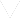تلفن: 88 94 08 47-50
آدرس مرکز تهران: خیابان استاد نجات الهی جنوبی، بین سپند و شاداب، کوچه نوید، پلاک 23
کلیک کنید
سمینار استانداردهای جدید حسابداری ایران
جزئیات این همایش

پادکست شماره 10 - معادله حسابداری

Listen to this Audio:

PACT Radio Accountant 10 - Basic Accounting Equation or Formula

Have you ever heard of the basic accounting equation or basic accounting formula? And do you know what this is? This equation is really, really important. In fact, all accounting entries are derived from this. If you really understand it, the rest of accounting is much easier. If you don't, you'll probably find the subject pretty hard. So listen up...

The whole of accounting is based on a single equation:

ASSETS = EQUITY + LIABILITIES

Accounting = Balance

3Save

The word equation comes from the word equal.

For any equation, one side always equals another. Also, equations can be made out of anything.

For example:

1 Orange = \$0,50

House = Walls + Doors + Windows + Roof

1 week = 7 days

So what does the basic accounting equation or basic accounting formula mean? Well, in order to answer that question we need to look at what each of the terms in the equation mean...

Construction Vehicle

Assets

Assets are possessions of the business. They are things that add value to the business and will bring it benefits in some form. For example, furniture, machinery, vehicles, computers, stationery or cash.

Equity

Equity, or owner's equity, is the value of the assets that the owner owns. It is the value of the business assets that the owner can lay claim to.

Liabilities

Liabilities are basically debts. The amount of liabilities represents the value of the business assets that are owed to others. It is the value of the assets that people outside the business can lay claim to.

What the Basic Accounting Equation Means

In a nutshell, the accounting equation above shows us how much of the assets are owned by the owner (equity) and how much are owed to others (liabilities). It's as simple as that.

به اشتراک بگذارید:

فایل پیوست
Questions - Solutions.zip (232KB)
فرم ارسال نظراتبا عضویت در خبرنامه از رویدادهای جدیدمان با خبر شوید!
Copyright 2019 PACT Edu. All right reserved.
در خبرنامه حسابداران عضو شوید تا:
از همایش‎های رایگان حسابداران خبره مطلع ‎شوید.
فرصت‎های شغلی حسابداری برای شما ارسال شود.
در جریان کلاس‎های جدید و تخفیفات دوره‎ها قرار گیرید.
خبرنامه ما هر 10 روز یکبار برای اعضا ارسال می‎شود.